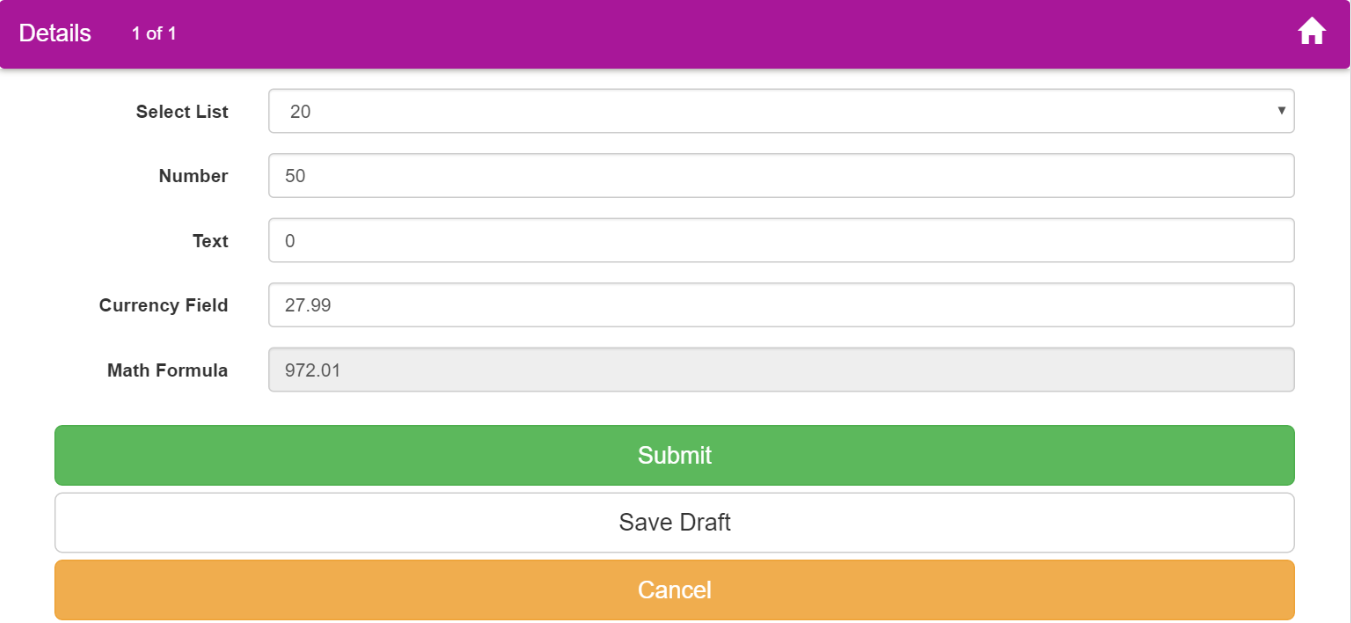# Math Formula Field - For Simple Mathematical Calculations

In this guide, we will explain how to use the Math Formula field on your Formyoula mobile forms.

The Formula field functionality is only available in the latest mobile application version 178. Please make sure to update your Formyoula iOS or Android app - http://support.formyoula.com/knowledgebase/articles/350088-where-to-find-the-formyoula-mobile-applications

Current Math Formula field version does not support Repeat group summary or totals.

The Math Formula field supports simple mathematical formulas for addition, subtraction, multiplication and division. Use field labels as merge values to build your expressions. We recommend using number and currency field types.

1.  Please drag & drop below form fields that will be used for the formula example. You can use Text, Number, Currency and Select fields.

Please note that you must use fields that contain number values only. Each field must contain a value for the formula to calculate the result, please use “0” values instead of leaving the fields blank.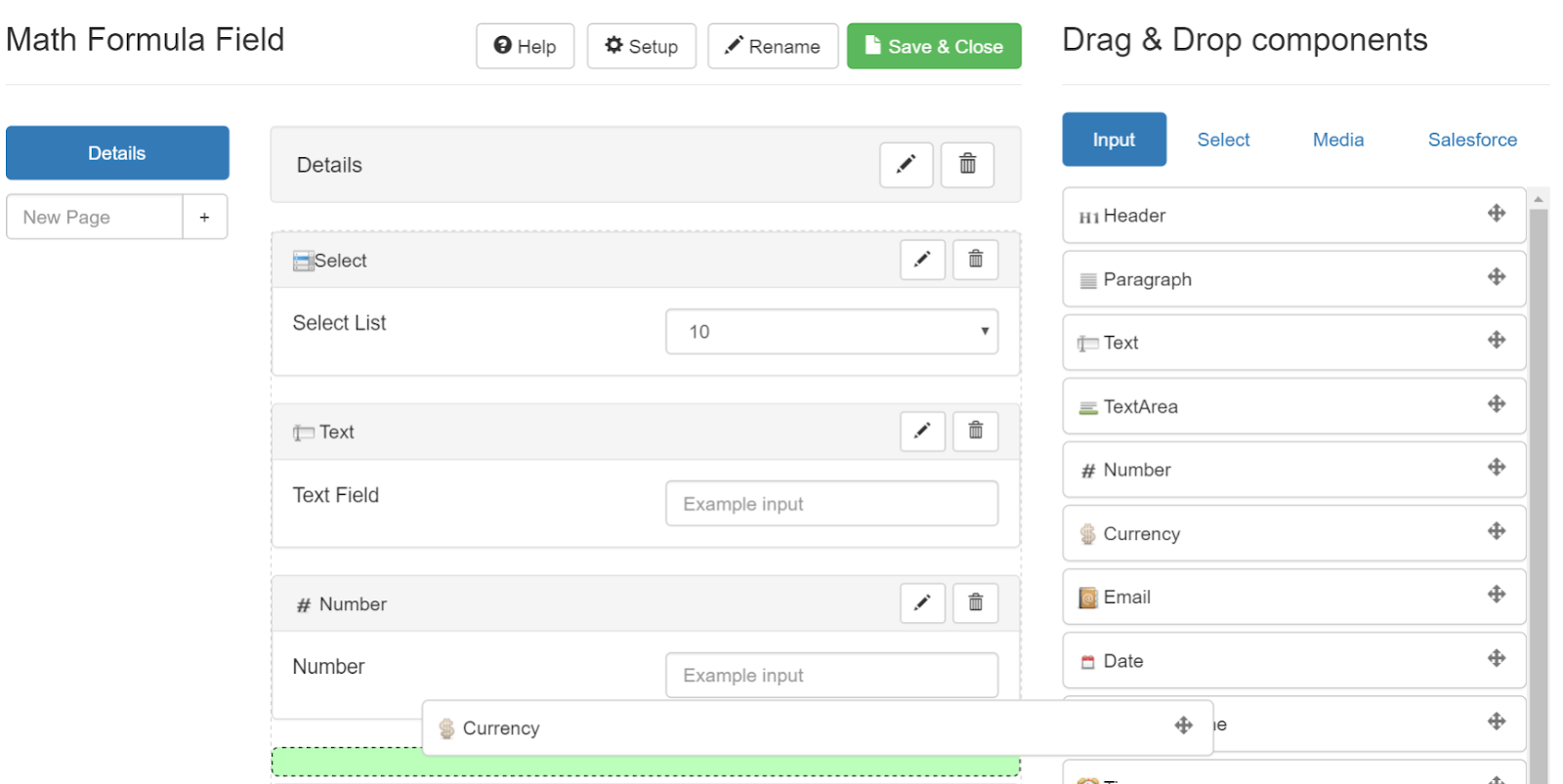2. Next please drag & drop the Math Formula field from the “Input” section.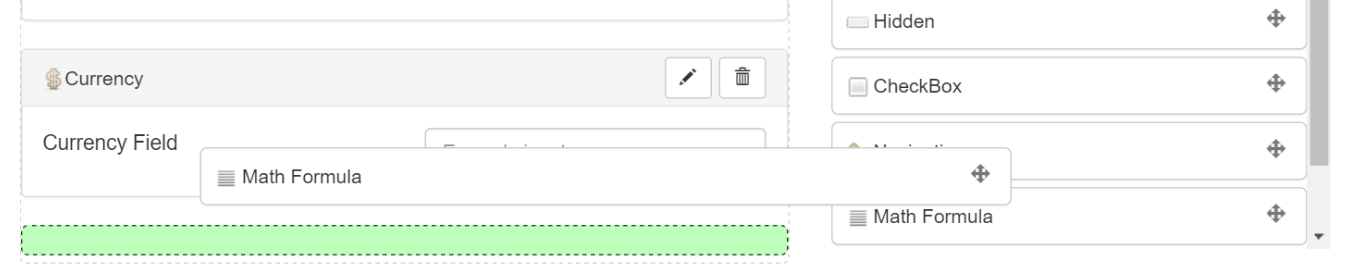3. Click on the “pencil” icon to edit the Math Formula field.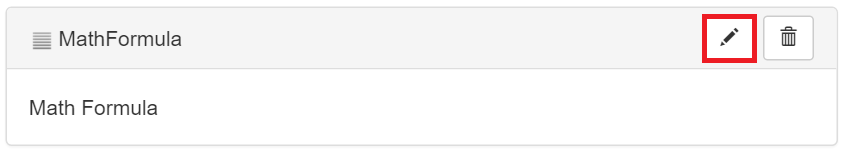4. Please use simple math equations under the “Math Formula Expression” textarea. To merge your form fields into the expression please use the form field labels surrounded by double curly braces. In this example we will multiply the “Select” field value with the “Number” field value then we will add the “Text” field value and will subtract it with the “Currency” field value.

You can use any number of form fields with any simple math equations.

• {{Field Label}} * {{Field Label}} + {{Field Label}} - {{Field Label}}

• {{Select List}} * {{Number}} + {{Text}} - {{Currency Field}}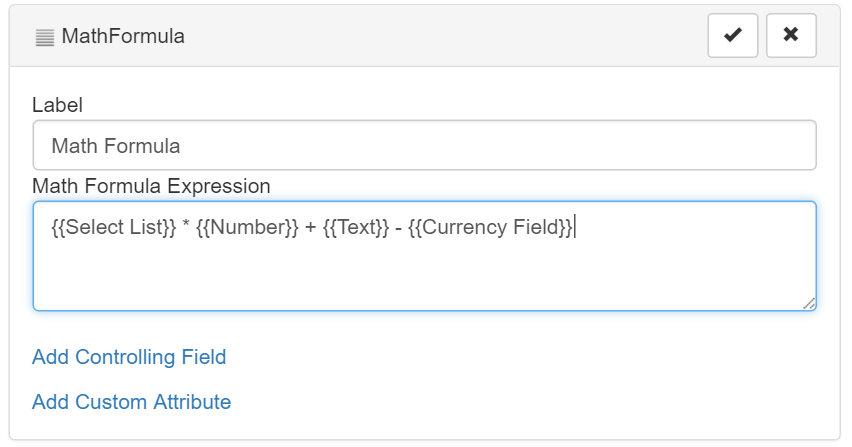5. When all equation fields are captured the Math Formula Field summary will be displayed the result in a read-only number. Formula fields can be mapped to Salesforce fields to record the calculated values on your Salesforce records.